Next: 15.1.3 The probability ranking Up: 15.1 Some Background on Previous: 15.1.1 Common design features

## 15.1.2 Evaluation measuers

• precision($BE,9gN((B), recall($B:F8=N((B)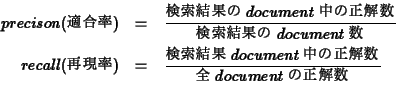• query $B$KBP$7$F(B,$B$9$Y$F$N(B document $B$r@52r$H=PNO$9$k(B system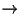recall 100%
• query $B$KBP$7$F(B $B@5$7$$(B document B?@(B1BDrEz(k(B systemprecison 100% B0lHLE*K(B trade off BN4X78(B • CUTOFF • N B0L(B BN(B precison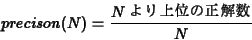• N Br(B cutoffBH$$$&(B (5,10,20,100) ($BNc(B Table 15.2)
• Uninterpolated average precision($BNc(B Figure 15.2) ranking 3 $B$N(B Uninterpolated average precison $B$O(B?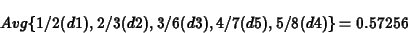• Interpolated average precison (11-point) • $B0lHLE*$JI>2A4p=(B, recall $B$r9MN8(B • recall 0% 10% 20%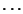100% (11 points) $B$K$*$1$k(B precision $B$NJ?6QCM(B
• recall $B$r(B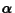$B$H$$&CMK8GDj7F(B B=N;~N(B precison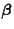Br5aak3HO0lHLE*K:Fq(B • B@52r,(B 2BD7+L5$$>l9g$O(B, recall $B$O(B 0,50,100% $B$N(B3 $BDL$j$7$+$J$$(B B@52r,==J,J8D?t"lP2DG=(B • precison B,C1D48:>/7J$$>l9g(B(Figure 15.2$B>e(B), $B$3$l$^$G$N:GBgCM$r(B precison $B$H$9$k(B
• interpolate (Figure 15.2$B2<(B) • precision-recall curves • $B0lHLE*$K1&2<$,$j(B • trade off $B$N4X78(B • F measures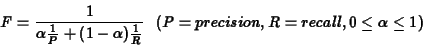• $B$3$l$i$NCM$O(B, $B8!:w(Bsystem $B$N@-G=I>2A$KMQ$$ilk(B, B7+7(B,B6vA3(B, system BK0MB87?(B query BEy,860xG(B, B@dBPE*JI>2A4p=HJj&kHO8BiJ$$(Bt$B8!Dj$J$I$rMQ$k(B
• $BM>CL(B TREC $B$N(B tool $B$K$3$l$i$NI>2ACM$r7W;;$9$k(B program $B$,$"$j$^$9(B

1999-08-03